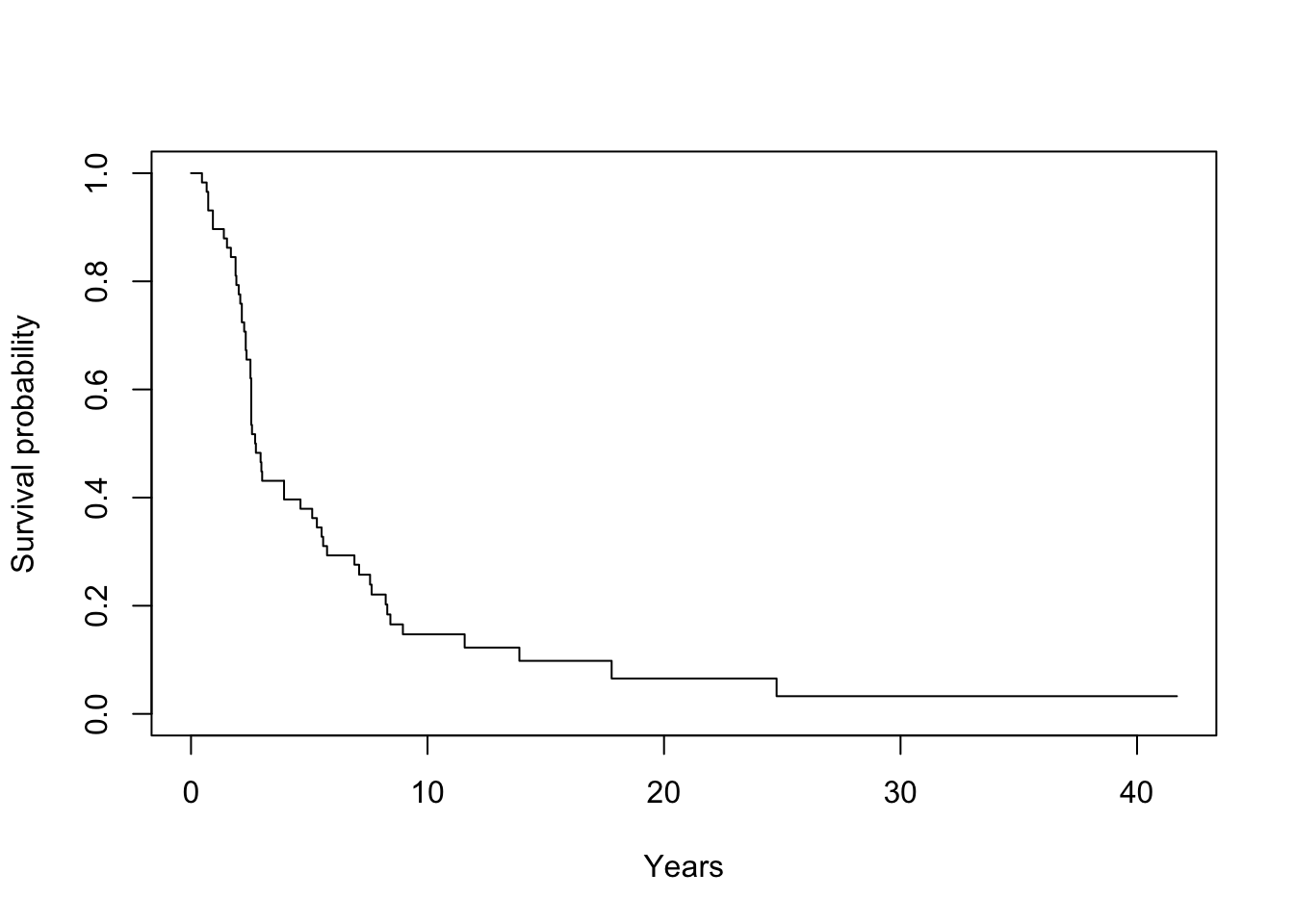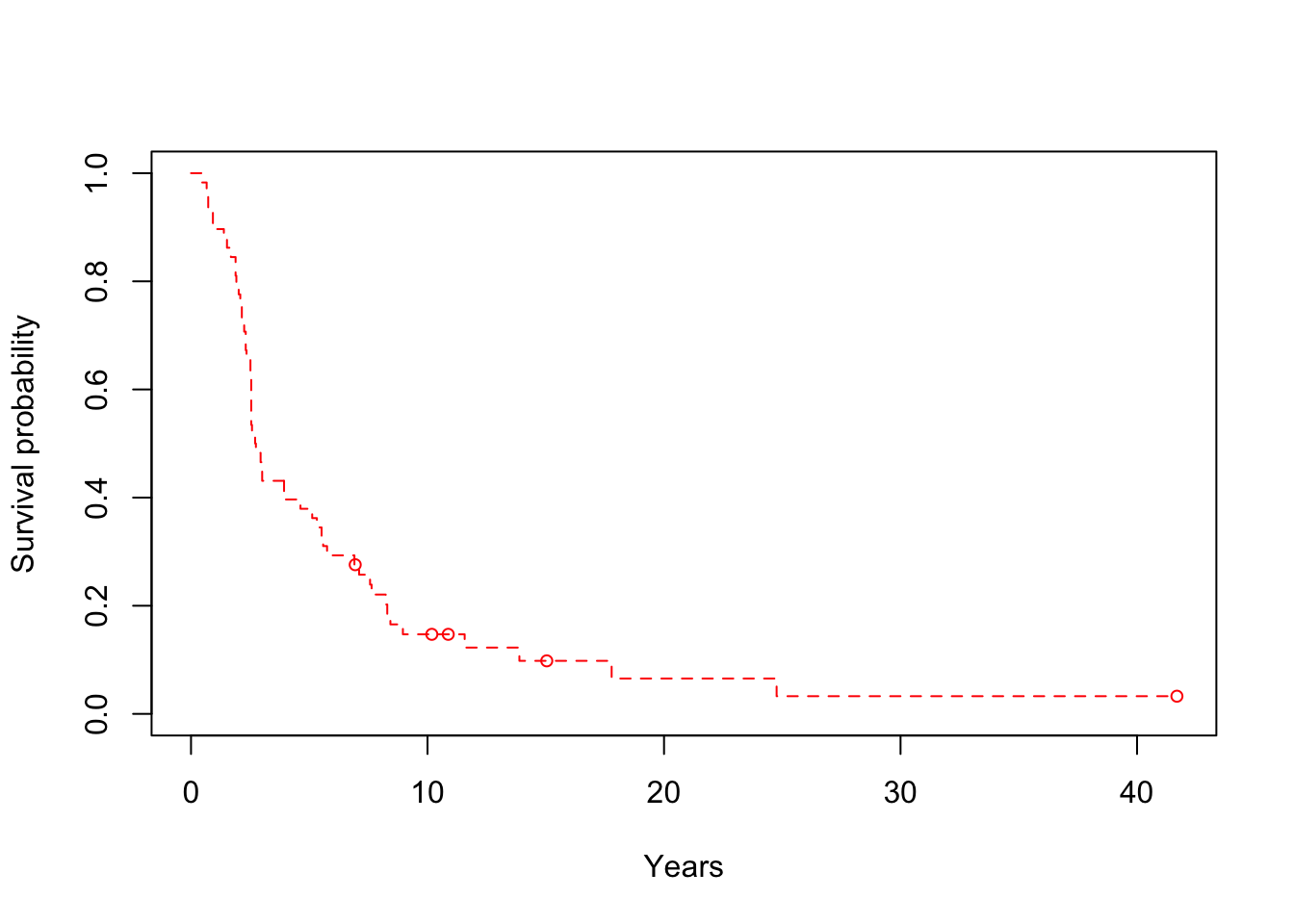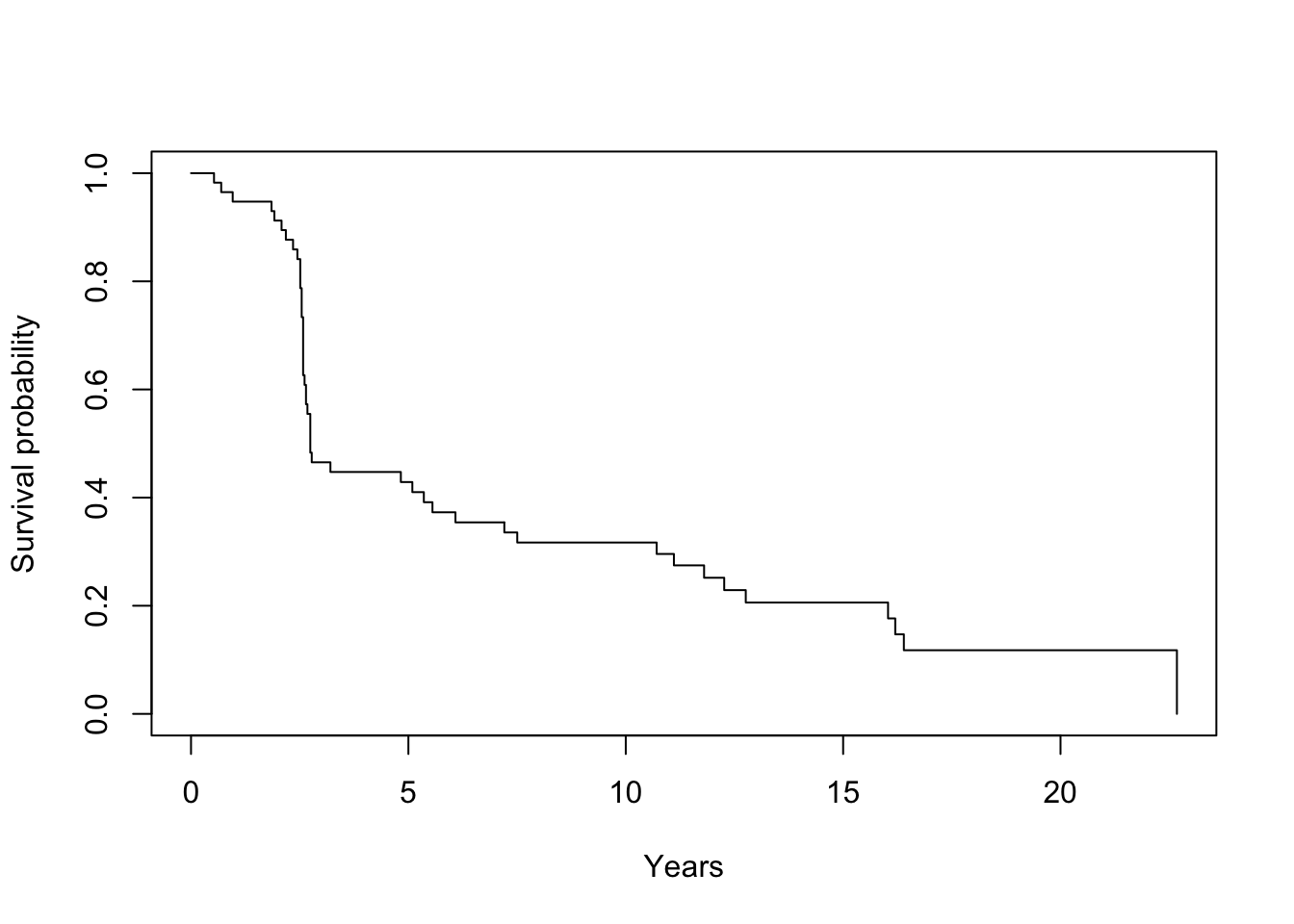# 1 General Information

This reduced piecewise exponential survival software implements the likelihood ratio test and backward elimination procedure in Han, Schell, and Kim (20121, 20142), and Han et al. (20163). Inputs to the program can be either times when events/censoring occur or the vectors of total time on test and the number of events. Outputs of the programs are times and the corresponding p-values in the backward elimination. Details about the model and implementation are given in Han et al. 2014. Adelson (20164) also mentioned the application of the method. This program can run in R version 3.2.2 and above.

# 2 Inputs and Outputs

## 2.1 Inputs

This software has one driver files RPEXEv1_2.R. Inputs to RPEXEv1_2.R include

• ‘EventTime’ = time, a vector having the times of the events occurred and of censoring. Note that ’EventTime’ is a required input.

• ‘Censor’ = censor, a vector with 0 or 1 indicating whether an observation is an event or censored. We use 0 to denote censoring and 1 to denote event. The length of time and censor are identical. Note that ’Censor’ is a required input.

• ‘CutTimes’ = cuttimes, a vector of the potential times where the piecewise exponential model is divided. Note that ‘CutTimes’ is an optional input. By default, cuttimes is a vector of times when the events occur.

• ‘Monotone’ = monotone, an indicator with values and meanings

• 0: no monotonic assumption;

• 1: assuming that the hazard is decreasing over time;

• 2: assuming that the hazard is increasing over time;

• 3: assuming that the hazard is monotonic;

• 4: assuming that the hazard is increasing and then decreasing;

• 5: assuming that the hazard is decreasing and then decreasing;

• 6: assuming that the hazard is increasing and then decreasing with the peak removed first;

• 7: assuming that the hazard is decreasing and then increasing with the peak removed first;

Note that ‘Monotone’ is an optional input. The default value of ‘Monotone’ is 0.

• ‘Criticalp’ = The critical (naive) p-value cutoff where all p-values in the backward elimination that are lower than this will be regarded as being significant. The prediction of the survival probability will be made on 100 equally spaced time points within the range of the event times based on the piecewise exponential estimate determined by all the changepoints. Default == -1 (equivalent to NA).

## 2.2 Outputs

• times: times to make the cuts

• pvalues: pvalues correspond to the times

• times_c: critical times to make the cuts

• pvalues_c: critical p-values that are smaller than the

• trend: trend information

• struct: structure information for multiple order restrictions

• changet: change point in time for umbrella alternatives.

# 3 Example with dataset data2

## 3.1 Load data and extract variables

``````library(RPEXE.RPEXT)
data(data2)
times = data2[,1]
censor = data2[,2]
group = data2[,3]

ID_nan = which(is.na(times))
times = times[-ID_nan]
censor = censor[-ID_nan]
group = group[-ID_nan]
armsA_ID = which(group == 1)
armsB_ID = which(group == 2)``````

## 3.2 Plot the data

``````# figure(1): Kaplan Meier curve of Arm A without indicating censored points
km(times[armsA_ID], censor[armsA_ID], 0)````````````# figure(2): Kaplan Meier curve of armA with censored points indicated
km_red(times[armsA_ID], censor[armsA_ID], 1)````````````# figure(3): Kaplan Meier curve of armB without indicating censored points
km(times[armsB_ID], censor[armsB_ID], 0)````````````# figure(4): Kaplan Meier curve of Arm B with censored points indicated
km_red(times[armsB_ID], censor[armsB_ID], 0)``````